# 5.1 Lab 5: ctft and its applications  (Page 4/4)

 Page 4 / 4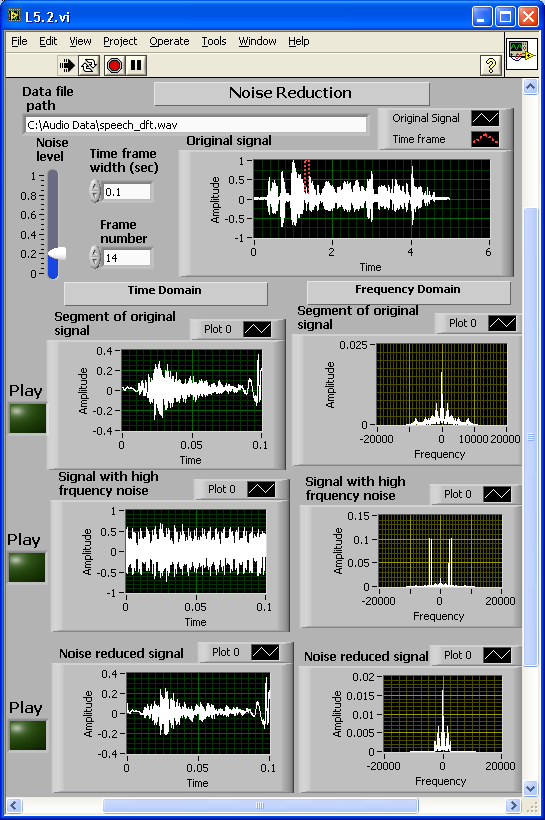Front Panel of a Noise Reduction System

## Amplitude modulation

In this section, we examine amplitude modulation and demodulation applications. For transmission purposes, signals are often modulated with a high-frequency carrier. A typical amplitude modulated signal can be described by

$x\left(t\right)={x}_{m}\left(t\right)\text{cos}\left({2\pi f}_{c}t\right)$

where ${x}_{m}\left(t\right)$ is called the message waveform, which contains the data of interest, and ${f}_{c}$ is the carrier wave frequency. Using the fact that

$\text{cos}\left({2\pi f}_{c}t\right)=\frac{1}{2}\left({e}^{{2\pi f}_{c}t}+{e}^{-{2\pi f}_{c}t}\right)=\frac{1}{2}\left({e}^{{\omega }_{c}t}+{e}^{-{\omega }_{c}t}\right)$

and the frequency shift property of CTFT, one can easily derive the CTFT ofto be

$X\left(\omega \right)=\frac{1}{2}\left({X}_{m}\left(\omega -{\omega }_{c}\right)+{X}_{m}\left(\omega +{\omega }_{c}\right)\right)$

At the receiver, some noisy version of this transmitted signal is received. The signal information resides in the envelope of the modulated signal, and thus an envelope detector can be used to recover the message signal.

[link] shows the completed block diagram of the amplitude modulation and demodulation system. In this example, use the combination of two sine waves to serve as a message signal. The signal is modulated with a high-frequency carrier, and some random noise is added. The frequency domain versions of the signals can also be observed using the function fft . As stated in Equation (3), the CTFT of the modulated signal is merely some frequency-shifted version of the original signal. In single sideband (SSB) modulation, only one side of the spectrum is transmitted due to symmetry. That is, just one side of the spectrum is taken and converted into a time signal using the function ifft .

[link] shows the completed front panel of this system. The Message signal, Modulated signal, Received signal (modulated signal with additional noise) and Demodulated signal are displayed in four waveform graphs in both the time and frequency domains.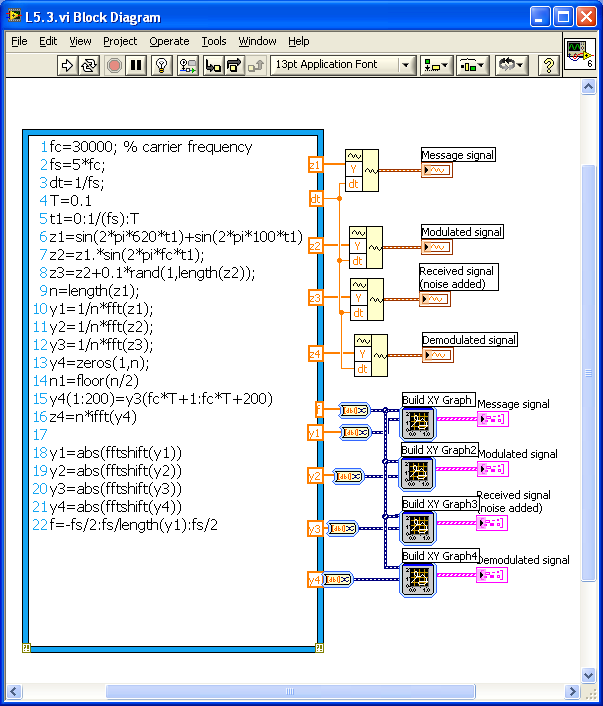Block Diagram of an Amplitude Modulation and Demodulation SystemFront Panel of an Amplitude Modulation and Demodulation System

## Lab exercises

Circuit Analysis

Find and plot the frequency response (both magnitude and phase spectrum) of each of the circuits shown in [link] . Set the values of R, L and C as controls.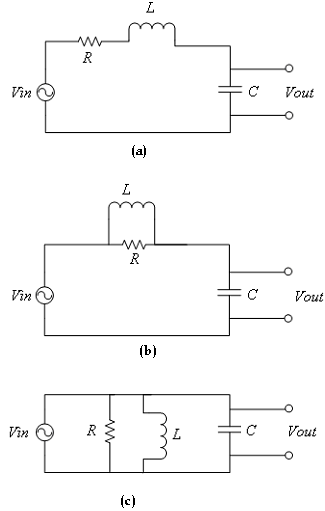Linear RLC Circuits

Insert Solution Text Here

Morse Coding

Consider a message containing some hidden information. Furthermore, to make it interesting, suppose the message contains a name. Assume that the message was coded using the amplitude modulation scheme as follows [link] :

$x\left(t\right)={x}_{\mathrm{m1}}\left(t\right)\text{cos}\left({2\pi f}_{1}t\right)+{x}_{\mathrm{m2}}\left(t\right)\text{cos}\left({2\pi f}_{2}t\right)+{x}_{\mathrm{m3}}\left(t\right)\text{cos}\left({2\pi f}_{3}t\right)$

where ${x}_{\mathrm{m1}}\left(t\right),{x}_{\mathrm{m2}}\left(t\right)$ and ${x}_{\mathrm{m3}}\left(t\right)$ are the (message) signals containing the three letters of the name. More specifically, each of the signals, ${x}_{\mathrm{m1}}\left(t\right),{x}_{\mathrm{m2}}\left(t\right)$ and ${x}_{\mathrm{m3}}\left(t\right)$ , corresponds to a single letter of the alphabet. These letters are encoded using the International Morse Code as indicated below :

$\begin{array}{ccccccccccc}A& \text{.}-& & H& \text{.}\text{.}\text{.}\text{.}& & O& ---& & V& \text{.}\text{.}\text{.}-\\ B& -\text{.}\text{.}\text{.}& & I& \text{.}\text{.}& & P& \text{.}--\text{.}& & W& \text{.}--\\ C& -\text{.}-\text{.}& & J& \text{.}---& & Q& --\text{.}-& & X& -\text{.}\text{.}-\\ D& -\text{.}\text{.}& & K& -\text{.}-& & R& \text{.}-\text{.}& & Y& -\text{.}--\\ E& \text{.}& & L& \text{.}-\text{.}\text{.}& & S& \text{.}\text{.}\text{.}& & Z& --\text{.}\text{.}\\ F& \text{.}\text{.}-\text{.}& & M& --& & T& -& & & \\ G& --\text{.}& & N& -\text{.}& & U& \text{.}\text{.}-& & & \end{array}$

Now to encode the letter A, one needs only a dot followed by a dash. That is, only two prototype signals are needed – one to represent the dash and one to represent the dot. Thus, for instance, to represent the letter A, set ${x}_{\mathrm{m1}}\left(t\right)=d\left(t\right)+\text{dash}\left(t\right)$ , where $d\left(t\right)$ represents the dot signal and $\text{dash}\left(t\right)$ the dash signal. Similarly, to represent the letter O, set ${x}_{\mathrm{m1}}\left(t\right)=3\text{dash}\left(t\right)$ .

Find the prototype signals $d\left(t\right)$ and $\text{dash}\left(t\right)$ in the file morse.mat on the book website. After loading the file morse.mat

>>load morse

the signals $d\left(t\right)$ and $\text{dash}\left(t\right)$ can be located in the vectors dot and dash, respectively. The hidden signal, which is encoded, per Equation (4), containing the letters of the name, is in the vector $\text{xt}$ Let the three modulation frequencies ${f}_{1},{f}_{2}$ and ${f}_{3}$ be 20, 40 and 80 Hz, respectively.

• Using the amplitude modulation property of the CTFT, determine the three possible letters and the hidden name. (Hint: Plot the CTFT of $\text{xt}$ Use the values of $T$ and $\tau \text{au}$ contained in the file.)

• Explain the strategy used to decode the message. Is the coding technique ambiguous? That is, is there a one-to-one mapping between the message waveforms ( ${x}_{\mathrm{m1}}\left(t\right),{x}_{\mathrm{m2}}\left(t\right),{x}_{\mathrm{m3}}\left(t\right)\right)$ ) and the alphabet letters? Or can you find multiple letters that correspond to the same message waveform?

Insert Solution Text Here

Doppler Effect

The Doppler effect phenomenon was covered in a previous chapter. In this exercise, let us examine the Doppler effect with a real sound wave rather than a periodic signal. The wave file firetrucksiren.wav on the book website contains a firetruck siren. Read the file using the LabVIEW MathScript function wavread and produce its upscale and downscale versions. Show the waves in the time and frequency domains (find the CTFT). Furthermore, play the sounds using the LabVIEW function Play Waveform . [link] shows a typical front panel for this system.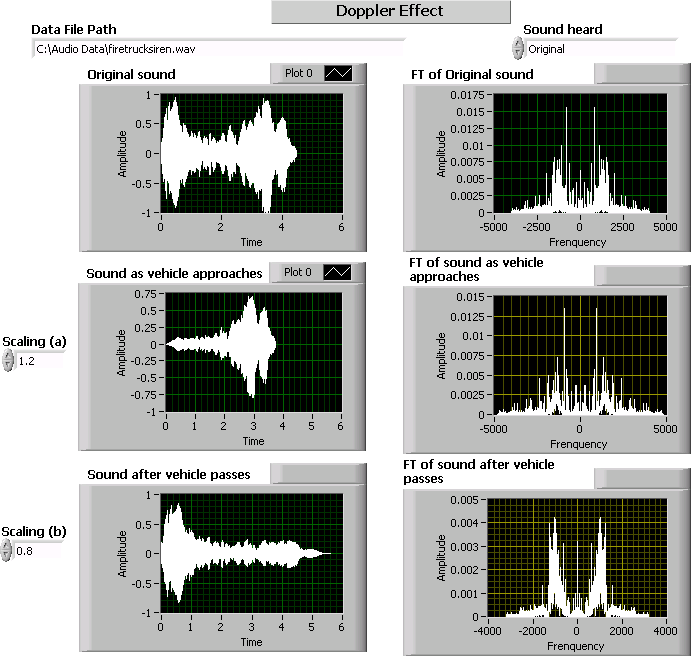Front Panel of Doppler Effect System

Insert Solution Text Here

Diffraction of Light

The diffraction of light can be described as a Fourier transform [link] . Consider an opaque screen with a small slit being illuminated by a normally incident uniform light wave, as shown in [link] .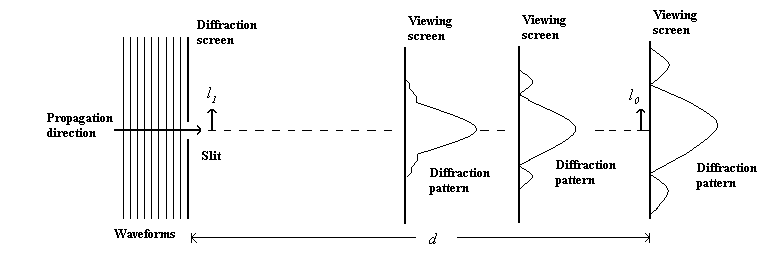Diffraction of Light

Considering that $d\text{>>}{\mathrm{\pi l}}_{{1}^{2}}/\lambda$ provides a good approximation for any ${l}_{1}$ in the slit, the electric field strength of the light striking the viewing screen can be expressed as [link]

${E}_{0}\left({l}_{0}\right)=K\frac{{e}^{j\left(2\pi d/\lambda \right)}}{\mathrm{j\lambda d}}{e}^{j\left(\pi /\mathrm{\lambda d}\right){l}_{{0}^{2}}}\underset{-\infty }{\overset{\infty }{\int }}{E}_{1}\left({l}_{1}\right){e}^{-j\left(2\pi /\mathrm{\lambda d}\right){l}_{0}{l}_{1}}{\text{dl}}_{1}$

where

${E}_{1}$ = field strength at diffraction screen

${E}_{0}$ = field strength at viewing screen

$K$ = constant of proportionality

$\lambda$ = wavelength of light

The above integral is in fact Fourier transformation in a different notation. One can write the field strength at the viewing screen as [link]

$\begin{array}{}\left(t\right)\\ {E}_{1}{}_{f\to {l}_{0}/\mathrm{\lambda d}}\\ \\ {E}_{0}\left({l}_{0}\right)=K\frac{{e}^{j\left(2\pi d/\lambda \right)}}{\mathrm{j\lambda d}}{e}^{j\left(\pi /\mathrm{\lambda d}\right){l}_{{0}^{2}}}\text{CTFT}\\ \end{array}$

The intensity $I\left({l}_{0}\right)$ of the light at the viewing screen is the square of the magnitude of the field strength. That is,

$I\left({l}_{0}\right)={\mid {E}_{0}\left({l}_{0}\right)\mid }^{2}$
1. Plot the intensity of the light at the viewing screen. Set the slit width to this range (0.5 to 5 mm), the wavelength of light $\lambda$ to this range (300 to 800 nm), and the distance of the viewing screen $d$ to this range (10 to 200 m) as controls. Assume the constant of proportionality is ${\text{10}}^{-3}$ , and the electric field strength at the diffraction screen is 1 V/m.
2. Now replace the slit with two slits, each 0.1 mm in width, separated by 1 mm (center-to-center) and centered on the optical axis. Plot the intensity of light in the viewing screen by setting the parameters in part (1) as controls.

Insert Solution Text Here

#### Questions & Answers

Is there any normative that regulates the use of silver nanoparticles?
Damian Reply
what king of growth are you checking .?
Renato
What fields keep nano created devices from performing or assimulating ? Magnetic fields ? Are do they assimilate ?
Stoney Reply
why we need to study biomolecules, molecular biology in nanotechnology?
Adin Reply
?
Kyle
yes I'm doing my masters in nanotechnology, we are being studying all these domains as well..
Adin
why?
Adin
what school?
Kyle
biomolecules are e building blocks of every organics and inorganic materials.
Joe
anyone know any internet site where one can find nanotechnology papers?
Damian Reply
research.net
kanaga
sciencedirect big data base
Ernesto
Introduction about quantum dots in nanotechnology
Praveena Reply
what does nano mean?
Anassong Reply
nano basically means 10^(-9). nanometer is a unit to measure length.
Bharti
do you think it's worthwhile in the long term to study the effects and possibilities of nanotechnology on viral treatment?
Damian Reply
absolutely yes
Daniel
how to know photocatalytic properties of tio2 nanoparticles...what to do now
Akash Reply
it is a goid question and i want to know the answer as well
Maciej
characteristics of micro business
Abigail
for teaching engĺish at school how nano technology help us
Anassong
Do somebody tell me a best nano engineering book for beginners?
s. Reply
there is no specific books for beginners but there is book called principle of nanotechnology
NANO
what is fullerene does it is used to make bukky balls
Devang Reply
are you nano engineer ?
s.
fullerene is a bucky ball aka Carbon 60 molecule. It was name by the architect Fuller. He design the geodesic dome. it resembles a soccer ball.
Tarell
what is the actual application of fullerenes nowadays?
Damian
That is a great question Damian. best way to answer that question is to Google it. there are hundreds of applications for buck minister fullerenes, from medical to aerospace. you can also find plenty of research papers that will give you great detail on the potential applications of fullerenes.
Tarell
what is the Synthesis, properties,and applications of carbon nano chemistry
Abhijith Reply
Mostly, they use nano carbon for electronics and for materials to be strengthened.
Virgil
is Bucky paper clear?
CYNTHIA
carbon nanotubes has various application in fuel cells membrane, current research on cancer drug,and in electronics MEMS and NEMS etc
NANO
so some one know about replacing silicon atom with phosphorous in semiconductors device?
s. Reply
Yeah, it is a pain to say the least. You basically have to heat the substarte up to around 1000 degrees celcius then pass phosphene gas over top of it, which is explosive and toxic by the way, under very low pressure.
Harper
Do you know which machine is used to that process?
s.
how to fabricate graphene ink ?
SUYASH Reply
for screen printed electrodes ?
SUYASH
What is lattice structure?
s. Reply
of graphene you mean?
Ebrahim
or in general
Ebrahim
in general
s.
Graphene has a hexagonal structure
tahir
On having this app for quite a bit time, Haven't realised there's a chat room in it.
Cied
what is biological synthesis of nanoparticles
Sanket Reply
how did you get the value of 2000N.What calculations are needed to arrive at it
Smarajit Reply
Privacy Information Security Software Version 1.1a
Good
Got questions? Join the online conversation and get instant answers!
Jobilize.com Reply

### Read also:

#### Get the best Algebra and trigonometry course in your pocket!

Source:  OpenStax, An interactive approach to signals and systems laboratory. OpenStax CNX. Sep 06, 2012 Download for free at http://cnx.org/content/col10667/1.14
Google Play and the Google Play logo are trademarks of Google Inc.

Notification Switch

Would you like to follow the 'An interactive approach to signals and systems laboratory' conversation and receive update notifications?ByByBy